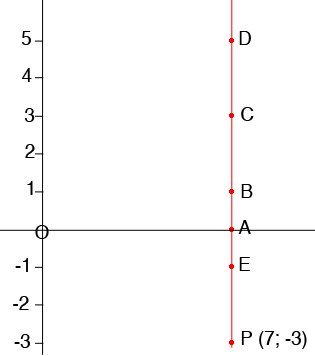SEARCH HOMEMath Central Quandaries & QueriesQuestion from Kagiso, a student: The equation for a vertical line that goes through the point (7; -3) is?Hi,

I sketched the Cartesian plane marking the X-axis, the Y-axis, the vertical line through P (7, -3)and I labeled other points on the line, A, B, C, D and E.What are the co-ordinates of A, B, C, D and E? What is the common feature of these co-ordinates? Write this fact algebraically. This is the equation of the line.

PennyMath Central is supported by the University of Regina and the Imperial Oil Foundation.